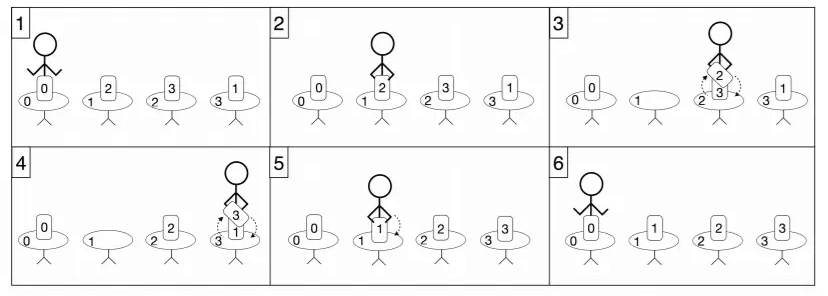# #325. 【IOI2017】Ancient Books

Aryan 从桌子 $s$ 开始重排这些古书。他希望在做完重排工作之后再回到同一张桌子上。由于这些古书非常珍贵，在任何时间，他手持的古书都不能超过一本。在重排古书的过程中，Aryan 将会做一系列的操作。每个操作只能是以下其中之一：

• 如果他手上没有书，而他所在的桌子上恰好有一本书时，他可以拿起这本书。
• 如果他手上有一本书，而他所在的桌子上恰好有另一本书时，他可以把手上的书和桌子上的书进行交换。
• 如果他手上有一本书，而他所在的桌子上没有书时，他可以把手上的书放到这个桌子上。
• 他可以走到任何一张桌子前。当他进行这个操作时，他手上可以拿一本书。

### 实现细节

 long long minimum_walk(std::vector<int> p, int s)

$p$ 是一个长度为 $n$ 的数组。初始阶段在桌子 $i$ 上的古书需要被 Aryan 移到桌子 $p[i]$ 上（对于所有 $0\leq i < n$）。

$s$ 是初始阶段 Aryan 所在桌子的编号，同时也是重排好所有古书之后他应该在的位置。

### 例子• 走到桌子 $1$ 处并且拿起桌上的书。这本书应该要被放到桌子 $2$ 上。
• 然后，他走到桌子 $2$ 处，并且把他手上的书和桌子上的书进行交换。现在他新拿到手上的书应该被放到桌子 $3$ 上。
• 然后，他走到桌子 $3$ 处，并且把他手上的书和桌子上的书进行交换。现在他新拿到手上的书应该被放到桌子 $1$ 上。
• 然后，他走到桌子 $1$ 处，并且把他手上的书放到桌子上。
• 最后，他回到桌子 $0$ 处。 注意，桌子 $0$ 上的书已经在正确的位置，即桌子 $0$ 上，因此 Aryan 不需要把它拿起来。在这个方案中，他的总行走距离是 $6$ 米。这是一个最优解；因此，函数应该返回 $6$。

### 数据范围

• $1\leq n\leq 1 \ 000\ 000$
• $0\leq s\leq n-1$
• 数组 $p$ 包含 $n$ 个从 $0$ 到 $n-1$（含）的不同整数。

$1$ $4$ $0$ $12$
$2$ $1000$ $10$
$3$ $1\ 000\ 000$ $28$
$4$ $1000$ $n-1$ $20$
$5$ $1\ 000\ 000$ $30$

### 评分程序样例

• 第 $1$ 行：$n\ s$
• 第 $2$ 行：$p\ p\ \cdots\ p[n-1]$